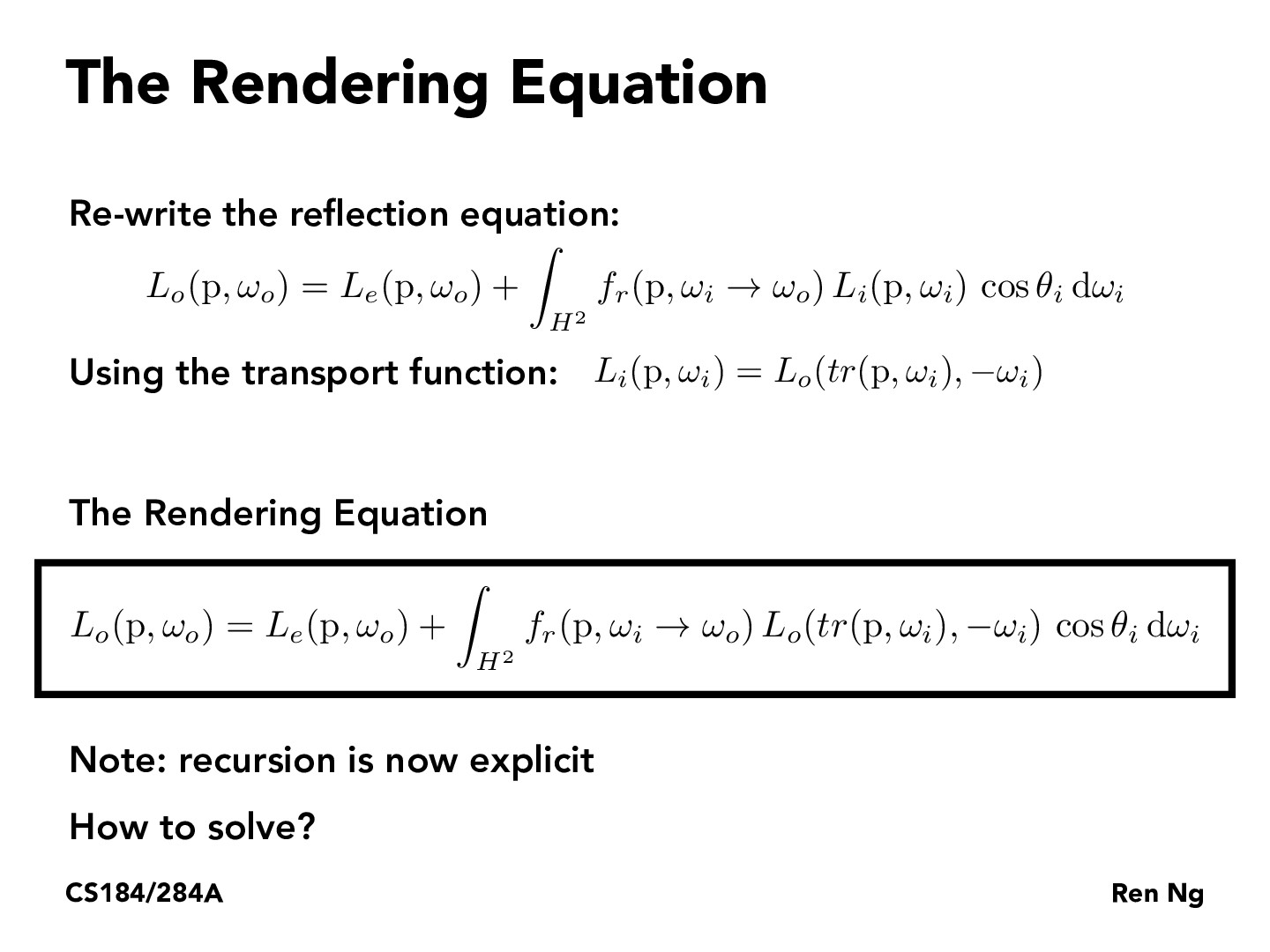You are viewing the course site for a past offering of this course. The current offering may be found here.Lecture 13: Global Illumination and Path Tracing (30)arjunsrinivasan1997

How are we able to substitute the transport function into our rendering equation to express it in the form as it is at the bottom of the slidego-lauren

@arjun If you look carefully, we see that the $L_i(p, \omega_i)$ term in the first equation of the slide is replaced by $L_0(tr(p, \omega_i), - \omega_i)$ in the boxed equation. This is exactly the equation we derived using the transport function. $tr()$ here is the transport function.

You must be enrolled in the course to comment